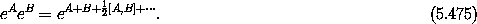## 5.11  Exponential Identities

The composition of Lie transforms can be written as products of exponentials of Lie derivative operators. In general, Lie derivative operators do not commute. If A and B are non-commuting operators, then the exponents do not combine in the usual way: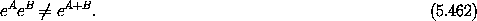So it will be helpful to recall some results about exponentials of non-commuting operators.

We introduce the commutator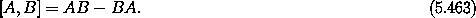The commutator is bilinear and satisfies the Jacobi identity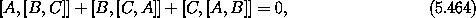which is true for all A, B, and C.

We introduce a notation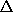A for the commutator with respect to the operator A: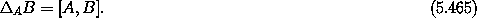In terms ofthe Jacobi identity is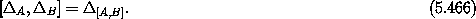An important identity is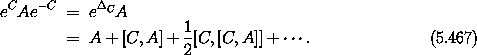We can check this term by term.

We see that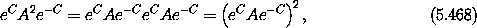using e-C eC = I, the identity operator. Using the same trick, we find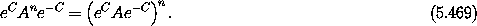More generally, if f can be represented as a power series then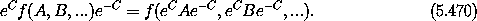For instance, applying this to the exponential function yields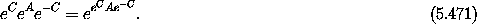Using equation (5.467), we can rewrite this as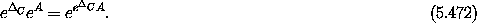Exercise 5.30.  Commutators of Lie derivatives

a.  Let W and W' be two phase-space state functions. Use the Poisson-bracket Jacobi identity (3.92) to show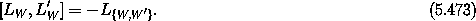b.  Consider the phase-space state functions that give the components of the angular momentum in terms of rectangular canonical coordinates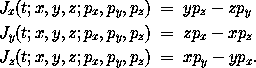Show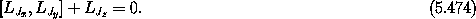c.  Relate the Jacobi identity for operators to the Poisson-bracket Jacobi identity.

Exercise 5.31.  Baker-Campbell-Hausdorff
Derive the rule for combining exponentials of non-commuting operators: